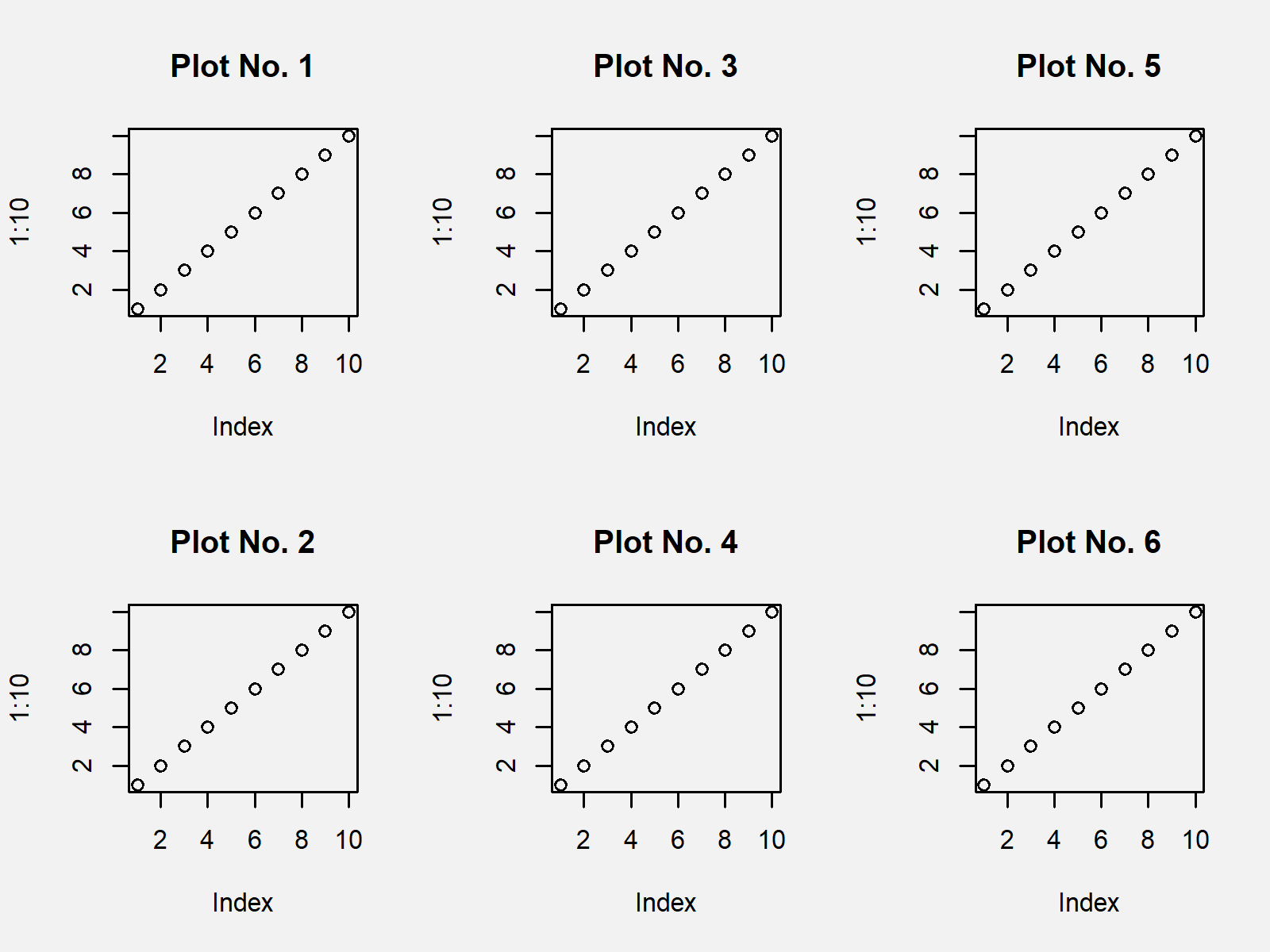# layout Function in R (3 Examples) | Arrange Grid of Plots in Base R

This tutorial illustrates how to specify a graphic layout using the layout() function in the R programming language.

It’s time to dive into the examples.

## Definition & Basic R Syntax of layout Function

Definition: The layout R function specifies complex plot arrangements.

Basic R Syntax: You can find the basic R programming syntax of the layout function below.

`layout(position_matrix)                                   # Basic R syntax of layout function`

In the remaining tutorial, I’ll illustrate in three examples how to apply the layout function in R programming. So keep on reading!

## Example 1: Define Plot Layout Using layout() Function in R

In Example 1, I’ll show how to use the layout function to create a plot matrix containing of three columns and two rows. First, we have to define a matrix, showing the positions of each of our plots:

```layout_matrix_1 <- matrix(1:6, ncol = 3)                  # Define position matrix
layout_matrix_1                                           # Print position matrix
#      [,1] [,2] [,3]
# [1,]    1    3    5
# [2,]    2    4    6```

The previous output illustrates the position matrix. The first plot will be shown at the upper left side, the second plot will be shown at the lower left side, the third plot will be shown in the upper middle position and so on…

Now, we have to apply the layout function to tell RStudio to arrange the upcoming plots as specified in our matrix:

`layout(layout_matrix_1)                                   # Specify layout`

Finally, we can draw our plots side-by-side as previously arranged:

```plot(1:10, main = "Plot No. 1")                           # One plot for each layout position
plot(1:10, main = "Plot No. 2")
plot(1:10, main = "Plot No. 3")
plot(1:10, main = "Plot No. 4")
plot(1:10, main = "Plot No. 5")
plot(1:10, main = "Plot No. 6")```The output of the previous R programming syntax is shown in Figure 1 – A plot arrangement showing six plots.

## Example 2: Create Layout with Empty Plot Positions

This Example shows how to leave some positions in our arranged graphic empty. For this, we have to specify a new position matrix were the empty positions are set to 0:

```layout_matrix_2 <- matrix(c(1, 2, 3, 0, 0, 4), ncol = 3)  # Define position matrix
layout_matrix_2
#      [,1] [,2] [,3]
# [1,]    1    3    0
# [2,]    2    0    4```

As you can see, the middle lower position and the upper right position were set to 0.

Now, we have to apply the layout function again:

`layout(layout_matrix_2)                                   # Specify layout`

And finally, we can draw our plot arrangement:

```plot(1:10, main = "Plot No. 1")                           # One plot for each layout position
plot(1:10, main = "Plot No. 2")
plot(1:10, main = "Plot No. 3")
plot(1:10, main = "Plot No. 4")```Figure 2 shows the output of the previous R programming syntax: Two positions of the plot matrix are not containing any plots.

## Example 3: Modify Widths & Heights of Plot Layout

In Example 3, I’ll illustrate how to modify the sizes of the plots in our plot matrix. We are using the same position matrix as in the previous example.

The layout function provides the widths and heights arguments. The widths argument should have the same length as the number of columns of our position matrix; and the heights argument should have the same length as the number of rows of our position matrix:

```layout(layout_matrix_2,                                   # Specify layout
widths = 1:3,
heights = 2:1)```

Now, let’s draw our plots accordingly:

```plot(1:10, main = "Plot No. 1")                           # One plot for each layout position
plot(1:10, main = "Plot No. 2")
plot(1:10, main = "Plot No. 3")
plot(1:10, main = "Plot No. 4")```As shown in Figure 3, we created a graph arrangement with different plot sizes with the previous R syntax.

## Video & Further Resources

I have recently published a video instruction on my YouTube channel, which explains the R code of this article. You can find the video tutorial below.

Please accept YouTube cookies to play this video. By accepting you will be accessing content from YouTube, a service provided by an external third party.If you accept this notice, your choice will be saved and the page will refresh.

In addition to the video, you might want to read the related articles on https://www.statisticsglobe.com/. I have published numerous articles already.

In summary: In this post you learned how to apply the layout() function in R programming. Let me know in the comments section, if you have additional questions and/or comments.

Subscribe to the Statistics Globe Newsletter